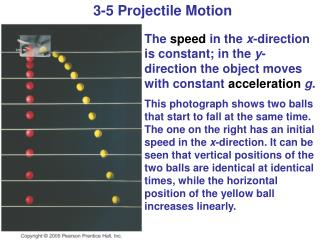# 3-5 Projectile Motion - PowerPoint PPT PresentationDownload Presentation3-5 Projectile Motion

3-5 Projectile Motion
Download Presentation## 3-5 Projectile Motion

- - - - - - - - - - - - - - - - - - - - - - - - - - - E N D - - - - - - - - - - - - - - - - - - - - - - - - - - -
##### Presentation Transcript

1. 3-5 Projectile Motion The speed in the x-direction is constant; in the y-direction the object moves with constant accelerationg. This photograph shows two balls that start to fall at the same time. The one on the right has an initial speed in the x-direction. It can be seen that vertical positions of the two balls are identical at identical times, while the horizontal position of the yellow ball increases linearly.

2. 3-5 Projectile Motion If an object is launched at an initial angle of θ0 with the horizontal, the analysis is similar except that the initial velocity has a vertical component.

3. 3-6 Solving Problems Involving Projectile Motion Projectile motion is motion with constant acceleration in two dimensions, where the acceleration is g and is down.

4. 3-6 Solving Problems Involving Projectile Motion • Read the problem carefully, and choose the object(s) you are going to analyze. • Draw a diagram. • Choose an origin and a coordinate system. • Decide on the time interval; this is the same in both directions, and includes only the time the object is moving with constant acceleration g. • Examine the x and y motions separately.

5. 3-6 Solving Problems Involving Projectile Motion 6. List known and unknown quantities. Remember that vx never changes, and that vy = 0 at the highest point. 7. Plan how you will proceed. Use the appropriate equations; you may have to combine some of them.

6. Example 3-4 A movie stunt driver on a motorcycle speeds horizontally off a 50.0 m high cliff. How fast must the motorcycle leave the cliff top to land on level grand below, 90.0 m from the base of the cliff where the cameras are? Ignore air resistance.

7. A football is kicked at an angle of 37.0 degrees with a velocity of 20.0 m/s. Calculate (a) the maximum height, (b) the time of travel before the football hits the ground, (c) how far away it hits the ground, (d) the velocity vector at the maximum height, and (e) the acceleration vector at the maximum height. Assume the ball leaves the foot at ground level, and ignore air resistance and rotation of the ball. Example 3-5

8. Example 3-5 (cont’d)

9. Conceptual Example 3-6 A child sits upright in a wagon which is moving to the right at constant speed. The child extends her hand and throws an apple straight upward (from her own point of view), while the wagon continues to travel forward (to the right) at constant speed. If air resistance is neglected, will the apple land (a) behind the wagon, (b) in the wagon, or (c) in front of the wagon?

10. Suppose the football in example 3-5 was a punt and left the punter’s foot at a height of 1.00 m above the ground. How far did the football travel before hitting the ground? Example 3-9

11. 3-7 Projectile Motion Is Parabolic In order to demonstrate that projectile motion is parabolic, we need to write y as a function of x. When we do, we find that it has the form: This is indeed the equation for a parabola.

12. 3-8 Relative Velocity We already considered relative speed in one dimension; it is similar in two dimensions except that we must add and subtract velocities as vectors. Each velocity is labeled first with the object, and second with the reference frame in which it has this velocity. Therefore, vWS is the velocity of the water in the shore frame, vBS is the velocity of the boat in the shore frame, and vBW is the velocity of the boat in the water frame.

13. 3-8 Relative Velocity In this case, the relationship between the three velocities is: (3-6)

14. Conceptual Example 3-10 A man in a small motor boat is trying to cross a river that flows due west with a strong current. The man starts on the south bank and is trying to reach the north bank directly north from his starting point. Should he (a) head due north, (b) head due west, (c) head in a northwesterly direction, or (d) head in a northeasterly direction?

15. Example 3-11 A boat’s speed in still water is vBW=1.85 m/s. If the boat is to travel directly across a river whose current has speed vWS=1.20 m/s, at what upstream angle must the boat head?

16. Summary of Chapter 3 • A quantity with magnitude and direction is a vector. • A quantity with magnitude but no direction is a scalar. • Vector addition can be done either graphically or using components. • The sum is called the resultant vector. • Projectile motion is the motion of an object near the Earth’s surface under the influence of gravity.

17. Homework - Ch. 3 • Questions #’s 1, 3, 5, 7, 9, 13, 17, 19 • Problems #’s 7, 11, 13, 21, 23, 25, 27, 29, 31, 37, 39, 41, 45